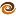# EmptyCrate

#### About Me | Contact Me | Training |ChaiScript.com | YouTube Channel

IIFE (Immediately-Invoked Function Expression) is a common tool used in JavaScript. The idea is to both define an anonymous function and call it in the same expression. The point is to produce a local scope for variables so they do not pollute the global scope.

This same technique can be deployed in C++ to lead to cleaner, safer, more performant code when building up objects which require multiple steps to initialize.

Complete code example available as a gist

Take this C++98 example:

# Good Old C++98

``````std::vector<std::vector<std::string>> classic(const int num_vecs, const int vec_size)
{
std::vector<std::vector<std::string>> retval;

for (int i = 0; i < num_vecs; ++i)
{
std::vector<std::string> nextvec(vec_size);
for (int j = 0; j < vec_size; ++j)
{
nextvec[j] = "Some string that's a little bit longer than a short string ";
// plus whatever else needs to happen
}
retval.push_back(nextvec);
// do some other house keeping here
}

return retval;
}
``````

Timing: 3106445us

For the rest of the context `nextvec` is doing no good, just taking up space. Plus, we had to copy it into `retval`, which does not lead to great performance.

So we think, “I know C++11, the solution here is to use `std::move`!”

# “Modern” C++11

``````std::vector<std::vector<std::string>> moved(const int num_vecs, const int vec_size)
{
std::vector<std::vector<std::string>> retval;

for (int i = 0; i < num_vecs; ++i)
{
std::vector<std::string> nextvec(vec_size);
for (int j = 0; j < vec_size; ++j)
{
nextvec[j] = "Some string that's a little bit longer than a short string ";
// plus whatever else needs to happen
}
retval.push_back(std::move(nextvec)); // this version requires extra bookkeeping to get the performance
// do some other house keeping here
// but we might be tempted to use nextvec, which is now is some unknown state
}

return retval;
}
``````

Timing: 1363530us

This is significantly better, but it has some issues I don’t like. First of all it seems like too much house keeping for C++. Second, it could be described as “dangerous” as now `nextvec` is in an unknown state. Using it could possibly crash?

We live in the land of RAII, certainly we can do better than this.

Perhaps we should take a step back and simply decompose the problem? After all, we know that the compiler is very good at optimizing return values.

# Problem Decomposition

``````std::vector<std::string> build_vector(const int vec_size)
{
std::vector<std::string> nextvec(vec_size);
for (int j = 0; j < vec_size; ++j)
{
nextvec[j] = "Some string that's a little bit longer than a short string ";
// plus whatever else needs to happen
}
return nextvec;
}

std::vector<std::vector<std::string>> function_call(const int num_vecs, const int vec_size)
{
std::vector<std::vector<std::string>> retval;

for (int i = 0; i < num_vecs; ++i)
{
retval.push_back(build_vector(vec_size));
}

return retval;
}
``````

This works quite well and doesn’t require any manual book keeping or leave any moved-out-of objects lying around.

Timing: 1015524us

We are now >3 times more efficient than the “standard” solution.

I’m still not 100% satisfied with the above, it seems a bit heavy handed to create a tiny function that’s used in exactly one place. Is there an C++11 features we might use to make this a little bit better?

# Immediately-Invoked Function Expression

``````std::vector<std::vector<std::string>> iife(const int num_vecs, const int vec_size)
{
std::vector<std::vector<std::string>> retval;

for (int i = 0; i < num_vecs; ++i)
{
retval.push_back([vec_size](){
std::vector<std::string> nextvec(vec_size);
for (int j = 0; j < vec_size; ++j)
{
nextvec[j] = "Some string that's a little bit longer than a short string ";
// plus whatever else needs to happen
}
return nextvec;
}());
// no extra bookkeeping
// no temptation to use the moved value
// no pollution of the local namespace with a 'bad' variable
}

return retval;
}
``````

The syntax is certainly not something C++ developers are used to seeing. The trailing `()` after the lambda expression is easy to miss.

However, this code captures the best of all of the options. It’s succinct. It’s fast. It doesn’t require any manual book keeping. It keeps the local scope clean.

Timing: 1009592us

It is slightly faster than the decomposed solution, but really in the margin of error for timing results.

# Final Note

Yes, this example is a bit contrived. If you truly need to initialize a bunch of multidimensional vector elements with the same value, use the built in constructors that accomplish that:

``````std::vector<std::vector<std::string>> smarter(const int num_vecs, const int vec_size)
{
return std::vector<std::vector<std::string>>(num_vecs, std::vector<std::string>(vec_size, "Some string that's a little bit longer than a short string "));
}
``````

Timing: 258654us

Using the proper API call to generate your data is now 12x faster than the original code.

1. Don’t get carried away with `std::move`
2. Consider using IIFE in C++, it could help make your code cleaner and safer
3. Don’t over-use IIFE, most developers are not used to seeing it and it could become confusing
4. Don’t forget to see if there’s already a standard library function that does what you need
My Videos

Related